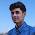## January 4, 2017

### Newton's First and Second Law of Motion

Newton's First Law of Motion
Law of inertia

An object at rest will remain at rest and an object that is moving at constant velocity will continue moving at constant velocity unless acted upon by an unbalanced force.

Newton's Second Law of Motion

The net force on an object is the product of its acceleration and its mass.

F = ma

F - Force
m - mass
a - acceleration

1N = 1kg  1m/s²1.1.